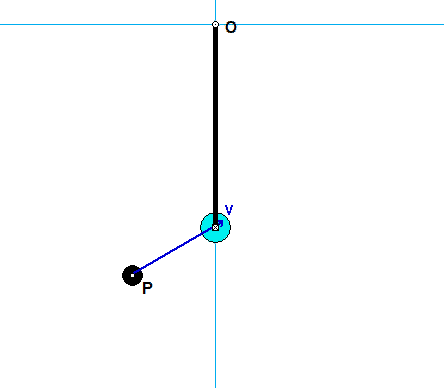# Homework H.4.TDiscussionYou are asked to investigate the dynamics of this system during the short time of impact of P with A.

• It is suggested that you consider a system made up of A+P+bar (make the system "big").
• Draw a free body diagram (FBD) of this system.
• For this system, linear momentum is NOT conserved since there are non-zero reaction forces at O.
• Furthermore, energy is NOT conserved since there is an impact of P with A during that time.
• From your FBD of the system, you see that the moment about the fixed point O is zero. What does this say about the angular momentum of the system about O during impact? (Answer: It is conserved!)

HINTS:

STEP 1 - FBD: Draw a SINGLE free body diagram (FBD) of the system of A+P+bar.
STEP 2 - Kinetics:  Consider the discussion above in regard to conservation of angular momentum about point O. Recall how to calculate the angular momentum about a point for a particle.
STEP 3 - Kinematics: At Instant 2, the P sticks to A: vP2 = vA2.
STEP 4 - Solve.